• 为了启用自动微分，PyTorch 跟踪所有涉及张量的操作，这些操作可能需要计算梯度(即 require _ grad is True)。这些操作被记录为有向图。Detach ()方法在一个被声明不需要梯度的张量上构造一个新视图，也就是说，它将...


目录
一、PyTorch分布式训练二、pytorch中常用的函数1.PyTorch .detach()2. softmax函数3. F.normalize
三、 pytorch 数据读取机制

一、PyTorch分布式训练
用两张显卡跑代码，使用pytorch模块DataParallel时出现的问题：
1
问题：AttributeError: ‘DataParallel’ object has no attribute ‘xxxx’ 解决 : model = model.module
2
问题：RuntimeError: grad can be implicitly created only for scalar outputs 报错行： loss.backward(retain_graph = True) 误差反向传播时出错 解决：loss.mean().backward(retain_graph = True) 将多卡得到的loss进行mean，求平均
3
问题：ValueError: only one element tensors can be converted to Python scalars 解决???
二、pytorch中常用的函数
1.PyTorch .detach()
为了启用自动微分，PyTorch 跟踪所有涉及张量的操作，这些操作可能需要计算梯度(即 require _ grad is True)。这些操作被记录为有向图。Detach ()方法在一个被声明不需要梯度的张量上构造一个新视图，也就是说，它将被排除在对操作的进一步跟踪之外，因此涉及这个视图的子图不会被记录。 当试图从计算图中删除一个张量时，应该使用detach()，并使用clone作为复制张量的一种方法，同时仍然保留该拷贝作为其来自的计算图的一部分。
eg1.
x = torch.ones((28, 28), dtype=torch.float32, requires_grad=True)
y=x**2
z=x**3

result = (y+z).sum()
result.backward()
#可以被正确微分得到梯度
#tensor([5., 5., 5., 5., 5., 5., 5., 5., 5., 5.])
torchviz.make_dot(result).render('Attached', format='png')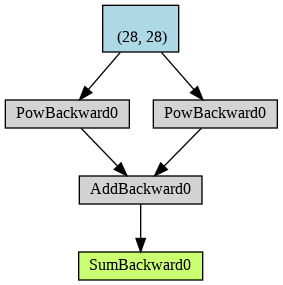eg2.
X = torch.ones((28, 28), dtype=torch.float32, requires_grad=True)
y = X**2
z = X.detach()**3

result = (y+z).sum()
result.backward()
#tensor([2., 2., 2., 2., 2., 2., 2., 2., 2., 2.])
#不再跟踪x**3的计算分支。这反映在结果的梯度中，它不再记录这一分支的贡献。
torchviz.make_dot(result).render('Attached', format='png')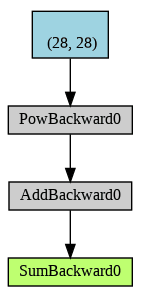2. softmax函数
第一步就是将模型的预测结果转化到指数函数上，这样保证了概率的非负性。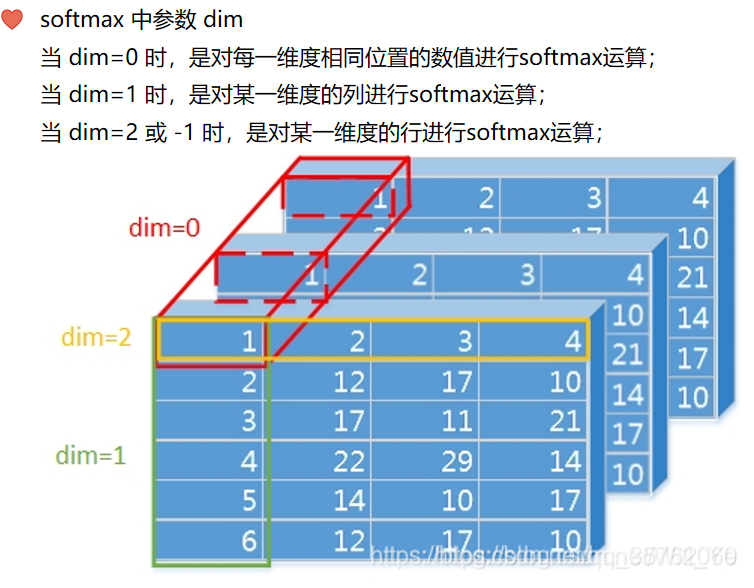3. F.normalize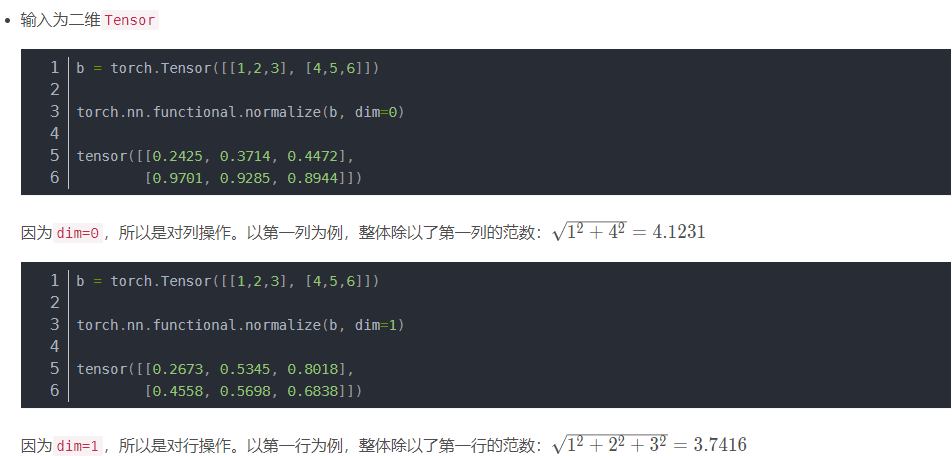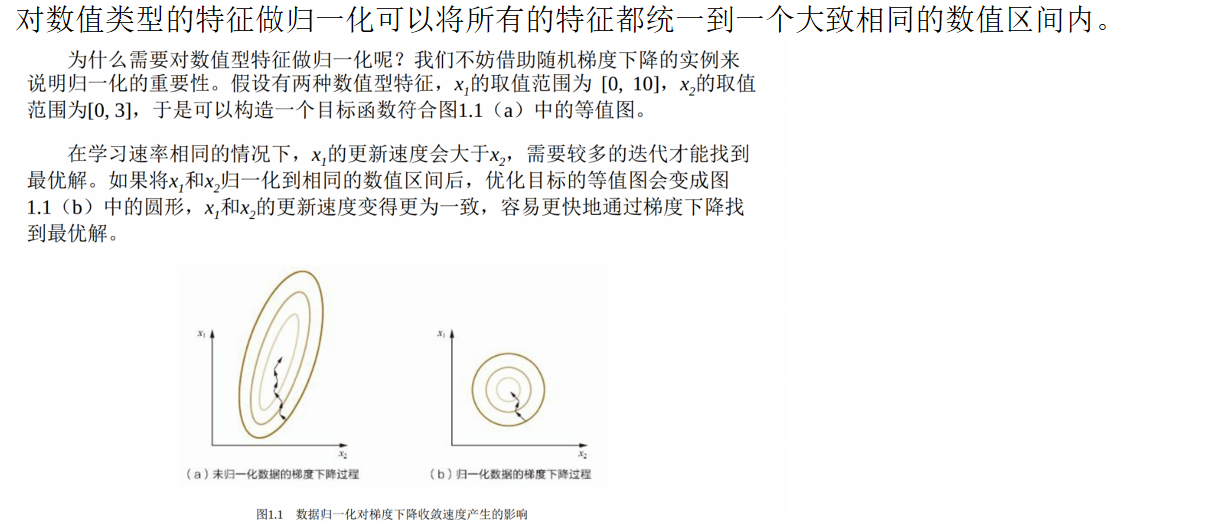三、 pytorch 数据读取机制
通过设置断点debug观察pytorch读取数据过程：
流程图如下所示：总结：Dataloder读取数据过程很复杂。虽然在构建数据集时候只需要实现Dataset的__len__方法和__getitem__方法，核心为__getitem__方法能根据下标读取数据集元素，通过debug之后，实际上整个过程需要跳转好几个函数才能最终读取数据。
展开全文python 深度学习
• numpy中默认浮点类型为64pytorch中默认浮点类型位32位 测试代码如下          numpy版本：1.19.2          ...
numpy中默认浮点类型为64位，pytorch中默认浮点类型位32位
测试代码如下          numpy版本：1.19.2          pytorch版本：1.2.0
In : import torch
In : import numpy as np
# 版本信息
In : "pytorch version: {}, numpy version: {}".format(torch.__version__, np.__version__)
Out: 'pytorch version: 1.2.0, numpy version: 1.19.2'

# numpy
In : dat_np = np.array([1,2,3], dtype="float")
In : dat_np.dtype
Out: dtype('float64')

# pytorch
In : dat_torch = torch.tensor([1,2,3])
In : dat_torch = dat_torch.float()
In : dat_torch.dtype
Out: torch.float32

展开全文numpy
• 量化是一种加速推理的技术，量化算子并且仅仅支持前向传递。Pytorch支持int8量化，相比于float32,模型的大小...pytorch提供了种量化模型的方法： 训练后动态量化：最简单的量化形式，权重被提前量化，激活在推理...

量化是一种加速推理的技术，量化算子并且仅仅支持前向传递。Pytorch支持int8量化，相比于float32,模型的大小减少4倍，内存要求减少4倍。与float32计算相比，对int8计算的硬件支持通常快2到4倍。
大多数情况下，模型需要以float32精度训练，然后将模型转换为int8。如今，PyTorch支持在具有AVX2支持或者更高版本的x86 CPU或者ARM CPU上运行量化运算符。
pytorch提供了三种量化模型的方法：

训练后动态量化：最简单的量化形式，权重被提前量化，激活在推理过程中被动态量化
训练后静态量化：最常用的量化形式，权重提前量化，并且基于观察校准过程中模型的行为来预先计算激活张量的比例因子和偏差。
量化意识训练：在极少数情况下，训练后量化不能提供足够的准确性，可以使用torch.quantization.FakeQuantize函数通过模拟量化来进行训练。

1. 前言
深度学习在移动端的应用越来越广泛，而移动端相对于GPU服务来讲算力较低并且存储空间也相对较小。基于这一点我们需要为移动端定制一些深度学习网络来满足我们的日常续需求，例如SqueezeNet，MobileNet，ShuffleNet等轻量级网络就是专为移动端设计的。但除了在网络方面进行改进，模型剪枝和量化应该算是最常用的优化方法了。剪枝就是将训练好的「大模型」的不重要的通道删除掉，在几乎不影响准确率的条件下对网络进行加速。而量化就是将浮点数（高精度）表示的权重和偏置用低精度整数（常用的有INT8）来近似表示&#
展开全文• 输入 conda install pytorch torchvision cuda80 -c soumith 参考资料 测试： $python >>> import pytorch 使用 资源 pytorch 预训练的模型 ... 安装 输入 conda install pytorch torchvision cuda80 -c soumith 参考资料 测试： $ python
>>> import pytorch

线性回归
torch.manual_seed的意义：产生相同的随机数，从而保证每次训练出来的模型是一样的 https://blog.csdn.net/zh515858237/article/details/83901179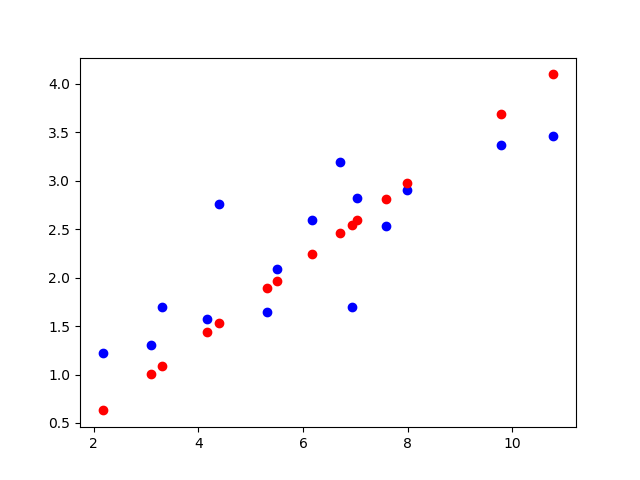# -*- coding:utf8 -*-
import torch
import numpy as np
import matplotlib.pyplot as plt

torch.manual_seed(2017)
# 读入数据 x 和 y
x_train = np.array([[3.3], [4.4], [5.5], [6.71], [6.93], [4.168],
[9.779], [6.182], [7.59], [2.167], [7.042],
[10.791], [5.313], [7.997], [3.1]], dtype=np.float32)

y_train = np.array([[1.7], [2.76], [2.09], [3.19], [1.694], [1.573],
[3.366], [2.596], [2.53], [1.221], [2.827],
[3.465], [1.65], [2.904], [1.3]], dtype=np.float32)
# 转换成 Tensor
x_train = torch.from_numpy(x_train)
y_train = torch.from_numpy(y_train)

# 定义参数 w 和 b
w = Variable(torch.randn(1), requires_grad=True) # 随机初始化
b = Variable(torch.zeros(1), requires_grad=True) # 使用 0 进行初始化

# 构建线性回归模型
x_train = Variable(x_train)
y_train = Variable(y_train)

def linear_model(x):
return x * w + b

# 计算误差
def get_loss(y_, y):

for e in range(10): # 进行 10 次更新
y_ = linear_model(x_train)
loss = get_loss(y_, y_train)
loss.backward()
w.data = w.data - 1e-2 * w.grad.data # 更新 w
b.data = b.data - 1e-2 * b.grad.data # 更新 b
print('epoch: {}, loss: {}'.format(e, loss))

y_ = linear_model(x_train)

plt.plot(x_train.data.numpy(), y_train.data.numpy(), 'bo', label='real')
plt.plot(x_train.data.numpy(), y_.data.numpy(), 'ro', label='estimated')
plt.show()
plt.legend()


卷积
# -*- coding:utf8 -*-
import numpy as np
import torch
from torch import nn
import torch.nn.functional as F
from PIL import Image
import matplotlib.pyplot as plt
im = Image.open('./cat.jpg').convert('L') # 读入一张灰度图的图片
im = np.array(im, dtype='float32') # 将其转换为一个矩阵
# 可视化图片
plt.imshow(im.astype('uint8'), cmap='gray')
plt.show()

# 将图片矩阵转化为 pytorch tensor，并适配卷积输入的要求
im = torch.from_numpy(im.reshape((1, 1, im.shape, im.shape)))  # (1,1,311, 481)

# 使用 nn.Conv2d
conv1 = nn.Conv2d(1, 1, 3, bias=False) # 定义卷积

sobel_kernel = np.array([[-1, -1, -1], [-1, 8, -1], [-1, -1, -1]], dtype='float32') # 定义轮廓检测算子 (3, 3)
sobel_kernel = sobel_kernel.reshape((1, 1, 3, 3)) # 适配卷积的输入输出
conv1.weight.data = torch.from_numpy(sobel_kernel) # 给卷积的 kernel 赋值

edge1 = conv1(Variable(im)) # 作用在图片上  ('edge1:', (1, 1, 309, 479))
print("edge1:",edge1.size())
edge1 = edge1.data.squeeze().numpy() # 将输出转换为图片的格式

plt.imshow(edge1, cmap='gray')
plt.show()

# 使用 F.conv2d
sobel_kernel = np.array([[-1, -1, -1], [-1, 8, -1], [-1, -1, -1]], dtype='float32') # 定义轮廓检测算子
sobel_kernel = sobel_kernel.reshape((1, 1, 3, 3)) # 适配卷积的输入输出
weight = Variable(torch.from_numpy(sobel_kernel))

edge2 = F.conv2d(Variable(im), weight) # 作用在图片上  ('edge2:', (1, 1, 309, 479))

print("edge2:",edge2.size())
edge2 = edge2.data.squeeze().numpy() # 将输出转换为图片的格式

plt.imshow(edge2, cmap='gray')
plt.show()

资源
pytorch 预训练的模型 https://pytorch.org/docs/stable/torchvision/models.html  pytorch 中文文档 https://ptorch.com/docs/1/torch-nn 
展开全文• pytorch安装 分步： 一、升级nvidia显卡。...、多python管理，一开始用是32位python3.7.2，后面下载了一个643.7.1。 具体步骤。 大概就是： 1、下载下来改python3.7.1的python.exe和pythonw.exe，改成熟悉python
• 1、Pytorch 简介 Pytorch 是一个基于 torch 的 python 开源机器学习库，主要用于自然语言处理等应用程序。说到这了就不得不介绍一下 torch ,它是有大量机器学习算法支持的科学计算框架，是与 Numpy 类似的张量...
• Pytorch一小时教程 pytorch是什么 它是一个基于python的科学计算库，致力于为两类用户提供服务： 一些想要找到Numpy搭建神经网络替代品的用户； 寻找一个可提供极强的可拓展性和运行速度的深度学习研究平台； 让...python ai 深度学习
• 一定要在64操作系统安装，因为32位系统不支持PyTorch。 1.1.安装Anaconda：Anaconda3-5.2.0-Windows-x86_64.exe 打开开始菜单中的“Anaconda3 (64-bit)”->“Anaconda Prompt”,以下所有命令都在其中执行。 ...
• ## PyTorch笔记1-PyTorch简介

千次阅读 多人点赞 2017-11-03 21:18:10
本系列笔记为莫烦PyTorch视频教程笔记 github源码PyTorch简介PyTorch的安装PyTorch的安装十分简单，根据PyTorch官网，对系统选择和安装方式等灵活选择即可。这里新建一个Python3的虚拟环境后，然后在虚拟环境下用pip...
• From and thanks to: github jcjohnson/pytorch-examples  本文通过自包含的示例介绍了PyTorch的基本概念，jcjohson的这些实例可以很好地帮助理解PyTorch与numpy、TensorFlow等之间的关系，以及其自己的概念和设计...
• ## Pytorch API备忘

千次阅读 2018-09-11 14:49:05
之前一直用tensorflow和keras，最近在看一些CV领域的paper，发现相关的开源代码很多是pytorch实现的，于是打算学下pytorch。 以下内容主要来于《深度学习入门之Pytorch》这本书。... 32位浮点 torch.DoubleTen...
• @pytorch:RuntimeError: invalid argument 0: Sizes of tensors must match except in dimension 0. Got 1 a pytorch报错 https://discuss.pytorch.org/t/runtimeerror-invalid-argument-0/17919/5
• @[TOC]目录 ...32位浮点 torch.FloatTensor torch.cuda.FloatTensor 64浮点 torch.DoubleTensor torch.cuda.DoubleTensor 16半精度浮点 N/A torch.cuda.HalfTensor 8无符号整型 torch.ByteTenso
• ## PyTorch 1.3

千次阅读 2019-10-13 23:32:49
2019.10.10日，PyTorch 开发者大会在美国旧金山开幕，会上发布了PyTorch 1.3。这次更新最大的亮点在于对移动设备的支持（Mobile Supported）、命名张量（Named Tensors），量化（Quantization），类型提升（Type ...
• PyTorch DataLoader 源代码 - 调试阶段 在本集中，我们将继续上集数据标准化的地方。只是这一次，我们将要调试代码，而不是编写代码，尤其是要调试PyTorch源代码，以查看规范化数据集时到底发生了什么。 调试...深度学习 神经网络 机器学习深度学习
• ## 莫烦 Pytorch

万次阅读 2020-03-21 09:35:39
Numpy Torch对比Pytorch import torch import numpy as np numpy_data = np.arange(6).reshape([2, 3]) # create a numpy torch_data = torch.from_numpy(numpy_data) # numpy--->torch tensor2array ...
• ## Pytorch TensorRT

千次阅读 2020-03-29 15:55:44
Pytorch通过保存为ONNX模型转TensorRT5 原创小关学长 最后发布于2019-04-15 16:08:35 阅读数 5506 收藏 展开 1 Pytorch以ONNX方式保存模型 def saveONNX(model, filepath): ...
•c++ 神经网络 深度学习 机器学习
• PyTorch简介 PyTorch 是 Torch 在 Python 上的衍生. 因为 Torch 是一个使用 Lua 语言的神经网络库,由于 PyTorch 采用了动态计算图（dynamic computational graph）结构，PyTorch 有一种独特的神经网络构建方法：...
• 蒲嘉宸的文章 - 知乎点击打开链接http://zhuanlan.zhihu.com/p/26871672Anaconda Windows
• 一、Pytorch介绍 1.1 什么是Pytorch PyTorch 是 Torch7 团队开发的，从它的名字就可以看出，其与 Torch 的不同之处在于 PyTorch 使用了 Python 作为开发语言。 PyTorch 既可以看做加入了 GPU 支持的 numpy，同时...
• pytorch环境 cuda10.1 torch1.4.0 certifi 2020.4.5.2 apex 0.1 cxxfilt 0.2.1 numpy 1.18.5 opencv-python 4.2.0.34 Pillow 7.1.2 pip 20.1.1 six 1.15.0 torch 1.4.0 torchvision 0.5.0 tqdm
• Pytorch官方英文文档：...疫情在家的这段时间，想系统的学习一遍Pytorch基础知识，因为我发现虽然直接Pytorch实战上手比较快，但是关于一些内部的原理知识其实并不是太懂，这样...finetune GPU使用
• Pytorch 基础入门 1.Pytorch常用包：[https://blog.csdn.net/qq_43270479/article/details/104077232] ...PyTorch系列 (): [pytorch数据读取：https://likewind.top/2019/02/01/Pytorch-dataprocess/] Pyt深度学习 神经网络
• conda install pytorch torchvision torchaudio cudatoolkit=10.2 -c pytorch 不带GPU的安装 conda install pytorch torchvision torchaudio cpuonly -c pytorch 安装之后在ipython中验证 import torch torch.__...深度学习
• 1、进入官网https://pytorch.org/ 2、选择要下载的版本 3、命令行输入 4、测试 import torch 成功 5、import torchvision 报错。 ModuleNotFoundError: No module named 'PIL' pip install pillow ...
• https://github.com/yunjey/pytorch-tutorial import torch import torch.nn as nn import numpy as np import matplotlib.pyplot as plt # Hyper-parameters input_size = 1 output_size = 1 num_epochs = 60 l....
• 文章目录pytorch学习笔记第一部分：pytorch入门1.1介绍pytorch1.2 安装1.3张量1.4自动求导机制1.5神经网络包nn和优化器optm1.6 数据的加载与预处理 第一部分：pytorch入门 1.1介绍pytorch PyTorch是一个基于Torch的...神经网络 深度学习
• Pytorch Note28 Pytorch的卷积模块 文章目录Pytorch Note28 Pytorch的卷积模块卷积层池化层 全部笔记的汇总贴：Pytorch Note 快乐星球 卷积网络在计算机视觉领域被应用得非常广泛，那么常见的卷机网络中用到的模块...卷积 网络 可视化 python 深度学习...